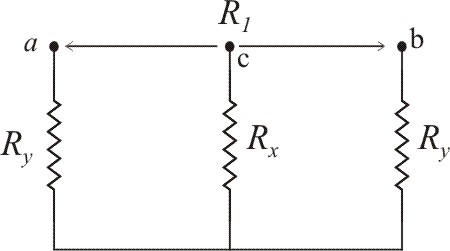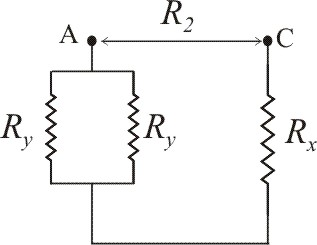Question# The student engineer of a campus radio station wishes to verify the effectivencess of the lightning rod on the antenna mast.

Other
ANSWEREDThe student engineer of a campus radio station wishes to verify the effectivencess of the lightning rod on the antenna mast. The unknown resistance $$\displaystyle{R}_{{x}}$$ is between points C and E. Point E is a "true ground", but is inaccessible for direct measurement because the stratum in which it is located is several meters below Earth's surface. Two identical rods are driven into the ground at A and B, introducing an unknown resistance $$\displaystyle{R}_{{y}}$$. The procedure for finding the unknown resistance $$\displaystyle{R}_{{x}}$$ is as follows. Measure resistance $$\displaystyle{R}_{{1}}$$ between points A and B. Then connect A and B with a heavy conducting wire and measure resistance $$\displaystyle{R}_{{2}}$$ between points A and C.Derive a formula for $$\displaystyle{R}_{{x}}$$ in terms of the observable resistances $$\displaystyle{R}_{{1}}$$ and $$\displaystyle{R}_{{2}}$$. A satisfactory ground resistance would be $$\displaystyle{R}_{{x}}{<}{2.0}$$ Ohms. Is the grounding of the station adequate if measurments give $$\displaystyle{R}_{{1}}={13}{O}{h}{m}{s}$$ and $$R_2=6.0$$ Ohms?2021-05-20

a) Two identical rods are driven into the ground at A and B, introducing an unknown resistance $$\displaystyle{R}_{{y}}$$. The procedure for finding the unknown resistance $$\displaystyle{R}_{{x}}$$ is as follows: measure resistance $$\displaystyle{R}_{{1}}$$ between points A and B. Then connect A and B with a heavy conducting wire, and measure resistance $$\displaystyle{R}_{{2}}$$ between points A and C. This shown in below figure.The equivalent resistance of series resistors $$\displaystyle{R}_{{y}}$$ and $$\displaystyle{R}_{{y}}$$ is
$$\displaystyle{R}_{{1}}={R}_{{y}}+{R}_{{y}}$$
$$\displaystyle={2}{R}_{{y}}$$From the above circuit $$\displaystyle{R}_{{y}}$$ and $$\displaystyle{R}_{{y}}$$ are connected in parallel. The equivalent resistance is
$$\displaystyle{R}_{{{e}{q}}}={\left[{\frac{{{1}}}{{{R}_{{y}}}}}+{\frac{{{1}}}{{{R}_{{y}}}}}\right]}^{{-{1}}}$$
$$\displaystyle={\frac{{{R}_{{y}}}}{{{2}}}}$$
And, $$\displaystyle{R}_{{{e}{q}}}$$ and $$\displaystyle{R}_{{x}}$$ are connected in series. Then the equivalent resistance is
$$\displaystyle{R}_{{2}}={R}_{{{e}{q}}}+{R}_{{x}}$$
$$\displaystyle={\frac{{{R}_{{y}}}}{{{2}}}}+{R}_{{x}}$$
From (1),
$$\displaystyle{R}_{{y}}={\frac{{{R}_{{1}}}}{{{2}}}}$$
Substitute this value in equation, we get
$$\displaystyle\therefore{R}_{{2}}={\frac{{{1}}}{{{2}}}}{\left({\frac{{{R}_{{1}}}}{{{2}}}}\right)}+{R}_{{x}}$$
$$\displaystyle={\frac{{{R}_{{1}}}}{{{4}}}}+{R}_{{x}}$$
$$\displaystyle\therefore{R}_{{x}}={R}_{{2}}-{\frac{{{R}_{{1}}}}{{{4}}}}$$
b) If the measurement give $$\displaystyle{R}_{{1}}={13}$$ Ohm and $$\displaystyle{R}_{{2}}={6.0}$$ Ohm
Then
$$\displaystyle{R}_{{x}}={R}_{{2}}-{\frac{{{R}_{{1}}}}{{{4}}}}$$
$$\displaystyle={\left({6.0}\ {O}{h}{m}\right)}-{\left({\frac{{{13}\ {O}{h}{m}}}{{{4}}}}\right)}$$
$$\displaystyle={2.75}$$ Ohm
$$\displaystyle\approx{2.8}$$ Ohm
Here satisfactory ground resistance would be $$\displaystyle{R}_{{x}}{<}{2.0}$$ Ohm. So $$\displaystyle{R}_{{x}}$$ is exceeds the limit of 2.0 Ohm
Therefore, the antenna is not adequate grounded.